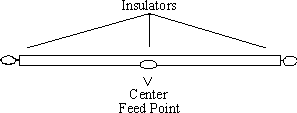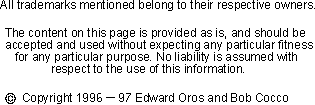Folded Dipoles

A variation of the dipole is an antenna called a folded dipole. It radiates like a dipole but sort of looks like a squashed quad.

Having a folded dipole does not mean that you have an antenna that is folded in half and so you obtain an antenna that now takes up half the space of a regular dipole. No, the antenna is still approximately the same length as a regular dipole. It is however, an antenna that has a wire folded back over itself, hence its name. Below is a picture of a folded dipole.The starting formula for the folded dipole calculation is the same as a dipole, 468 / Frequency (in MHz). Let's try an example: Design a folded dipole for the 40 meter band. The frequency that is chosen might be 7.15 MHz. Plugging this in to the formula (468 / 7.15) gives a folded dipole with a length of 65.45 feet. When I modeled a dipole on the computer at 30 feet, I came up with a length of 65.47 feet. When I added a second wire to make the folded dipole shown above, I designed the antenna with 1 inch spacing between the two wires. Note that this adds 1 more inch to each of the two antenna wires over that of a single wire dipole. This plus the fact that we are actually turning up the ends of the antenna, means that the horizontal length actually need to be a little shorter to be once again at resonance. The total length came to 64.38 feet, 1.09 feet shorter than the straight dipole. If you use a greater spacing, say 1 foot between the wires the length is 63.1 feet, 2.37 feet shorter. So be sure to shorten the antenna a bit or you'll find yourself operating lower down the band than you expected.

The feed point impedance is also modified by the second wire. Let's say the original dipole was 72 ohms. The step-up for a two wire folded dipole is 4 times which means 4 * 72 = ~288 ohms. (The computer shows 281 ohms on my example, but remember, we reduced the length slightly also.) This step up continues if you add more and more wires. A three wire antenna would provide a step-up of 9, and a four wire antenna provides a step-up of 16.

We can see why this step-up occurs by looking at the power formula P=(I*I) * R, this can be rewritten as R = P / (I*I). If the power to a regular dipole antenna was 100 watts and the current was 1.2 amps, we'd solve for R as R = 100 / (1.2*1.2), which is the same as R = 100 / 1.44, which is 69.44 ohms. In the folded dipole the wires are in parallel, the current must be divided between the two wires. The current in each is half and the total power has not changed, so now the formula is R = 100 / (.6*.6), which is the same as R = 100 / .36, which of course is 277.77 ohms, 4 times the normal dipole antenna.

So now you ask, why would anyone want an antenna with a feed point impedance of 277 ohms, my coax cable is 50 ohms!? Well let's say you wanted to feed the antenna, not with 50 ohm cable but with 300 ohm twin lead? Ah ha, now we have a decent match and a feed line that can also handle a higher SWR with low loss. You'd probably use a tuner (ATU) in the shack to match the 50 ohm radio to the 300 ohm feed line. You could also use the antenna on other bands with the tuner and have an efficient antenna system.

What are the drawbacks to the antenna? Well for one, the currents on each wire will begin to cancel each other out on even multiples of the cut frequency, so a 40 meter folded dipole should not be used on 14 MHz. On other bands even though the signal may cancel broad side to the antenna, you'll find that there is actually gain! This occurs about 45 degrees off broad side to the antenna. And this might make for interesting contacts.

Back to the Antenna Elmer